M403K First Midterm Exam
Given February 5, 2002

1. An explosive shell is shot out of a cannon. Its height above the ground (in feet) is given by the function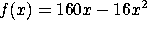, where x is the time (in seconds) since the firing.

a) Find the velocity of the shell as a function of time.

b) What is the shell's velocity at time x=4? At that time, is it heading up or down?

c) The shell will hit the ground again at time x=10. What will its velocity be at that time?

2. a) From the following table, estimate f'(3). Indicate clearly how you obtain your answer: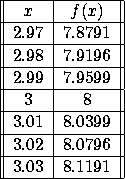b) You receive a secret message saying that the function in part (a) was really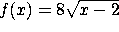. If this message is true, what should f'(3) equal? How does this compare to the answer you got in part (a)?

3. Evaluate the following limits, if they exist (or write DNE if they do not).

a)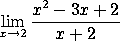b)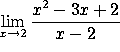c)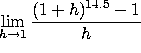[Note: This was a typo.  I had intended this question to ask about the limit as h goes to zero, not as h goes to one.]

d)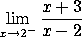4. Take the derivatives of the following functions with respect to x. You do not need to simplify your answers:

a)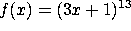b)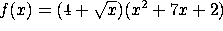c)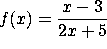d)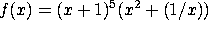5. Nimbus Racing Brooms, Ltd. has just released their finest model, the Nimbus 2002. (Available at Quality Quiddich Supplies and fine stores everywhere.) Their marketing department has determined that the demand function is x = 800 - 40p, where x is the number of brooms sold (per month) and p is the price (in gold galleons). The cost function is C(x)= 500 + 10x.

a) Find the price p(x) and the revenue R(x) as a function of x.

b) Compute the marginal cost, marginal revenue and marginal profit as a function of x.

c) The factory is currently producing x=300 brooms/month. To increase revenue, should the company increase or decrease production?

d) Likewise, if the company wants to increase profit, should it increase or decrease production?